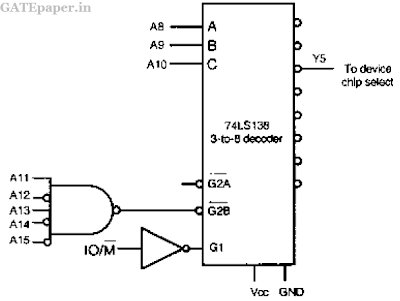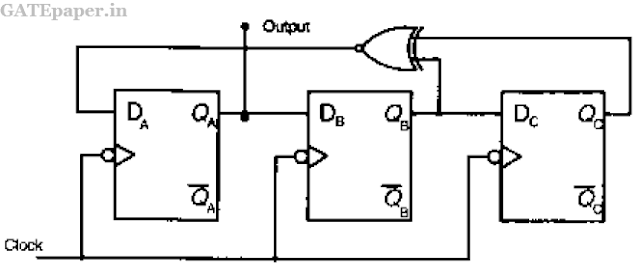### GATE 2010 ECE Video Solution on Digital Circuits (Digital Electronics)

1. Match the logic gates in Column A with their equivalents in Column B.a. P – 2, Q – 4, R – 1, S – 3
b. P – 4, Q – 2, R – 1, S – 3
c. P – 2, Q – 4, R – 3, S – 1
d. P – 4, Q – 2, R – 3, S – 1

2. For the output F to be 1 in the logic circuit shown, the input combination should bea. A = 1, B = 1, C = 0
b. A = 1, B = 0, C = 0
c. A = 0, B = 1, C = 0
d. A = 0, B = 0, C = 1

3. In the circuit shown, the device connected to Y5 can have address in the rangea. 2000 – 20FF
b. 2D00 – 2DFF
c. 2E00 – 2EFF
d. FD00 – FDFF

4. Assuming that all flip-flops are in reset condition initially, the count sequence observed at QA in the circuit shown isa. 0010111….
b. 0001011….
c. 0101111….
d. 0110100….

5. The Boolean function realized by the logic circuit shown isa. F = ∑m(0,1,3,5,9,10,14)
b. F = ∑m(2,3,5,7,8,12,13)
c. F = ∑m(1,2,4,5,11,14,15)
d. F = ∑m(2,3,5,7,8,9,12)

6. For the 8085 assembly language program given below, the content of the accumulator after the execution of the program is

3000   MVI A, 45H
3002   MOV B, A
3003   STC
3004   CMC
3005   RAR
3006   XRA B

a. 00H
b. 45H
c. 67H
d. E7H